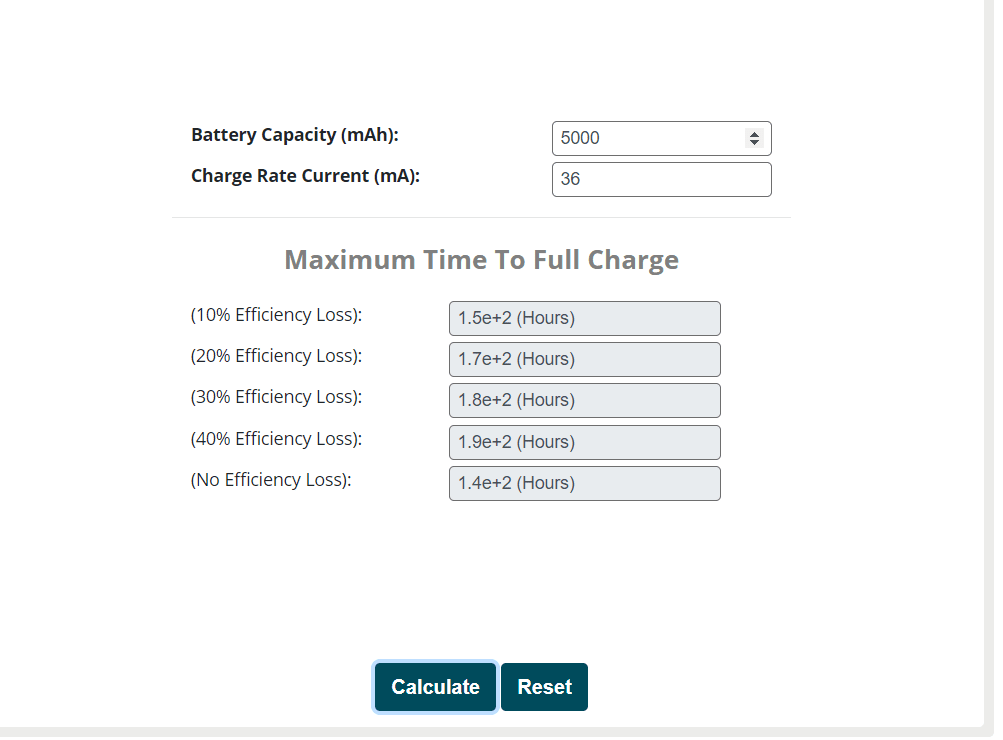# Home Solar Batteries: Calculate the Charge Time

Most of the peoples think that calculating the charging time for solar batteries is easy because they just have to divide the capacity of the batteries by the total power of the solar panels.

This is not as easy as the above facts explain. This usually because this calculation doesn’t include the loss of power during this charging or consuming process.

There are different possible reasons for the loss of powers including the following:

• The drop in the voltage of the solar panels or the expected power point is lower than the peak panel voltage
• The loss of energy due to the conversion of electrical energy into chemical energy. This includes the extra heating of the elements in solar panels which can create a huge loss of energy.
• Energy consumed in the circuit for lowering or boosting the voltage.

In this article, we will discuss how to calculate the charge time batteries that are attached to the solar panels at your home. Using these methods, you can calculate the charging time accurately and easily yourself.

## Types of batteries and method of calculating

Commonly, two types of batteries could be attached with the solar panels including:

• Lithium-Ion Battery

### 1. Charging time of Lithium-Ion battery with solar panels

The process of charging the battery of Lithium-Ion battery with solar panels slows down when the capacity reaches 90%.

This way, 90% is considered to be full in the sense of charging and storage.

You can use the following formula for calculating the battery charging time of your solar batteries:

(Battery capacity (watts/hour) / power of solar panel) x 2

This is formula calculates more accurately during the sky days rather than the perfect days. This is because the combination of the solar panels and the battery is more performing on sky days as compared to the sunny day.

Example:

Total power of Solar Panel = 6 Watt

Battery voltage = 3.7 v

Battery capacity = 4,000 mAh

Using formula, 14.8 / 6 x 2 = 4.9 h

In this calculation, the charge rate should be accurate because it can affect the calculation in a bad way.

### 2. Charging time of lead Acid battery with solar panels

The capacity of the lead-acid battery is nearly 50% but the rest 50% shouldn’t be discharged. This is because the rest 50% also contains efficiency as compared to the lithium batteries.

For calculation of the charging of this type of battery, you need to consume the capacity of the battery as the true capacity and this capacity will be considered in the calculation.

Here is the formula for this type of battery:

The capacity of Battery (Watts) x 2 / Power of the solar panel (Watts)

## Factors that affect the variation in the charge time

The biggest factor that affects the charge time of the solar panels for the batteries is the environmental factor.

The environment is the biggest reason for variation in charging time. This is because the solar panels that are directly facing the sun and its sunny day then it will give better charging time.

In sky days, although the charging time of the batteries is slow the loss of energy is also low.

## Easy way to calculate charging timeOne of the ways to calculate the charging time of solar batteries is mentioned above but there is an alternative that is quite a more accurate and faster way to calculate.

The online charge time calculator can help you to calculate the charging time of the calculator automatically.

However, the online calculator just requires some basic information like battery Capacity in (mAh) as well as the Charge Rate Current in (mA). Once you enter, it will give you’re the result of the required charging time to charge your batteries from solar panels.

Related – 7 Ways To Save Electricity at Home